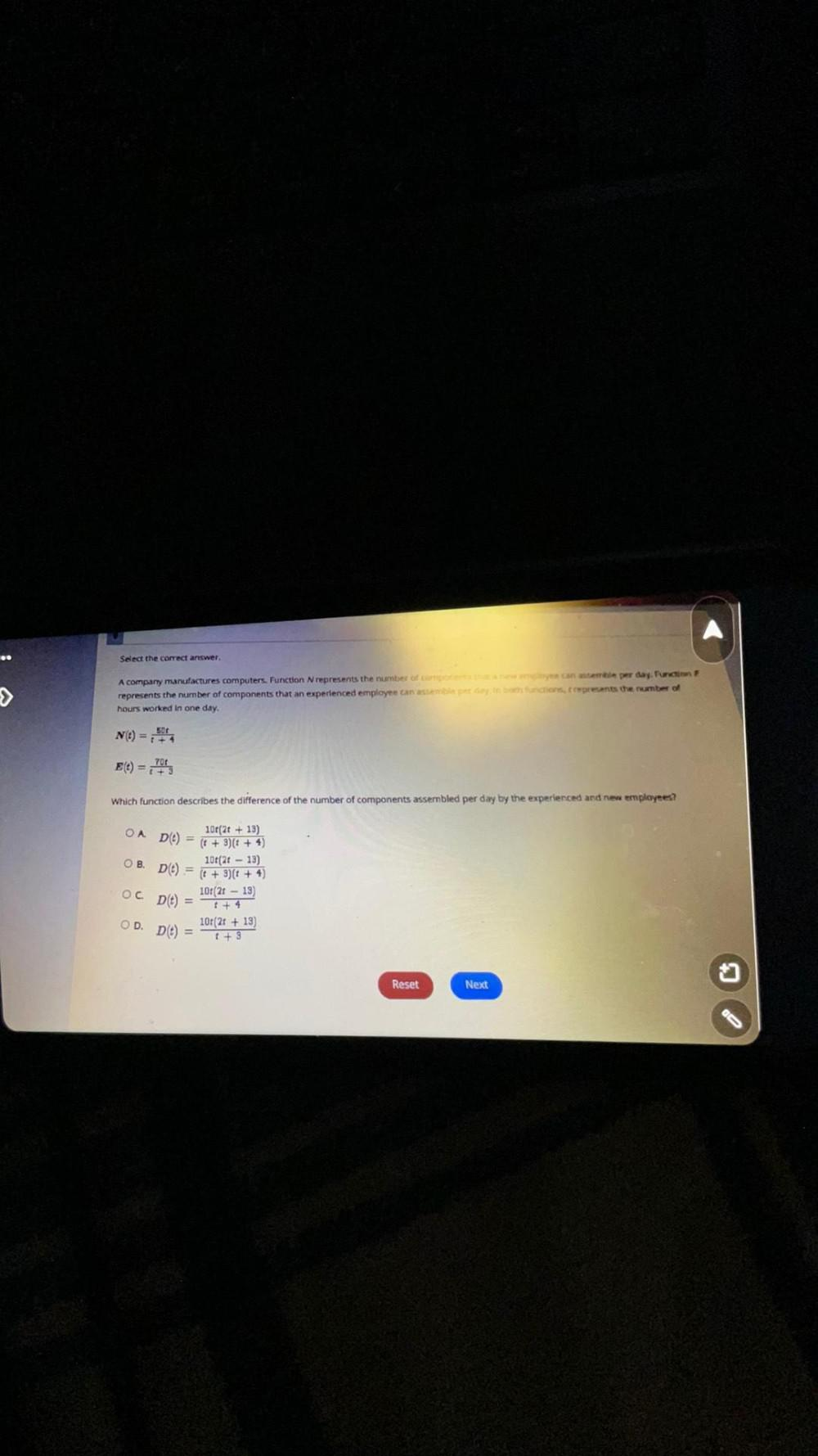Question:

# > Select the correct answer, A company manufactures computers. Function N represents the number of components that a new eyes ca> Select the correct answer, A company manufactures computers. Function N represents the number of components that a new eyes can assemble per day. Function F represents the number of components that an experienced employee can assemble per day fr bath functions, rrepresents the number of hours worked in one day. 50t N(t) = +4 E(t) = 70 Which function describes the difference of the number of components assembled per day by the experienced and new employees? 10r(2t+13) OA D() = (t + 3)(+4) 10t(213) OB. D(t)= (t + 3)(² + 4) 101 (2013) +4 OC D(t) = 10:(21+13) 1+3 OD. D(t) = Reset Next